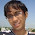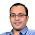Aug 22, 2009

Puzzle: Working Computer

Problem:
A room has n computers, less than half of which are damaged. It is possible to query a computer about the status of any computer. A damaged computer could give wrong answers. The goal is to discover an undamaged computer in as few queries as possible.

(Once again, posed by Gurmeet Singh Manku on his blog)

Solution:
Highlight the part between the * symbols for the answer.
* [Thanks to Gaurav Bubna for his solution]

Pair up all the computers. In every pair, test one comp with other. We have 3 possibilities - correct correct, correct wrong, wrong wrong. correct correct can only come if both comps are either correct or both wrong. From each such pair just keep one comp.

Note that: Since for wrong wrong pairs and correct wrong pairs, both working can never be the case, so, in correct correct pairs, number of working > no of damaged.

So, the property number of working > no of damaged is preserved in the next iteration.

Note that we reduce number of elements bt more than half. So, T(n) < T(n/2) + n, i.e T(n) < 2n

So, in less than 2n comparisons, we will be able to find a working computer :) *

1.Isme yeh kahan prove hua ki 2k is the minimum number of query required,

2.I am not able to prove that this is minimum.. All I am claiming is that I can always get a solution in 2n checks..

Can someone suggest a better solution? or a proof that this is the best solution..?

3.here is a solution in which in N-1 computers we would be able to find a working computer.
we will try to find a chain of computer (i1,i2,i3....im) such that each ij is queried about i(j+1) and answer is correct.Note that if such chain contains a working computer then last computer in the chain would be working.so query computer 1 about 2.If answer is yes query computer 2 about 3.continue till answer is correct.suppose at some point computer i when queried about computer j says wrong,in that case remove computer i and j from the chain,and continue query process by querying predecessor of i about successor of j.here note that when we remove computer i and j from the chain at-least one of them must be faulty.here for each computer accept the first one we are querying once.hence N-1 comparison in worst case.think about the best case using this algorithm.

4.great solution...

seems correct to me.... :)

5.Say, total m computers are there. Fix one of them (say, the m-th one). Ask each computer 1,2,3,4,........m (so, we ask the fixed computer too : this is allowed since the question mentions that any computer can tell about any computer} about this fixed computer.. Say k say 'fine' and m-1-k say 'damaged'. Thus we get two groups of computers: G1={all computers which say m-th one is fine} and G2= {all computers which say m-th one is damaged}. Then those computers are fine which belong to the group having more members (since, less than half are damaged)... i guess this works

1."Fix one of them" - Its not allowed!

Fraction Brainteaser

Source: Sent to me by Gaurav Sinha Problem: Siddhant writes a Maths test and correctly answers 5 out of 6 Arithmetic questions and 20...